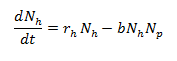Explore BrainMass

# Prey and Predator Diversity

Predator and prey dynamics are characteristic of an oscillating pattern which results from the co-evolution between these species. In the simplest form, predator and prey interactions are modelled using one predator population and one prey population.

Similar to competition for limiting resources, predation also acts as a force controlling prey population sizes. One difference between these two forms of control is that limiting resources act as a “bottom-up” control, whereas predation acts as a “top-down” control. This is because limited resources affect the bottom of the food chain first and then influence the subsequent levels.

Predation works the opposite way putting pressure on prey populations and therefore, contributing to their population’s decline. For prey populations to survive, they need to avoid predation and have access to food resources. Furthermore, through evolution prey develop traits to help them avoid predators more efficiently such as evolving better defense mechanisms or behavioural traits to signal to the population that predators are coming. In turn, predators evolve traits to overcome these barriers to predation.

The Lotka-Volterra predator-prey model provides a simplified, mathematical model for understanding these population dynamics. However, this model does have some assumptions:

1. This model assumes that prey will grow exponentially in the absence of predators.
2. It is assumed that the predator population grows at a rate equivalent to the rate at which predator’s convert prey to offspring. This basically means, how much dead prey is required for a predator to produce offspring which will become a new predator at a future time.
3. Predators can consume infinite amounts of prey and will starve in the absence of this one prey species being considered.
4. This model does not take environmental complexity into account.

In the following equations, snowshoe hares (h) represent the prey and foxes (f) represent the predator.

Equation for how the prey population grows:Variables:

rhNh = rate of prey growth (r = growth rate of the prey population, N = abundance of prey population)

b = attack efficiency

bNhNp = rate of prey decline

Equation for how the predator population grows:Variables:

c = conversion factor (how many dead prey it takes to produce a new predator)

b = attack efficiency

dp = per capita death rate of predators

N = abundance (Nh = abundance of prey, Np = abundance of predators)

Title Image Credit:flickr.com